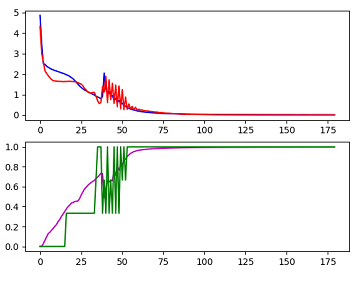# Jumpy validation graph

hi, i have a very jumpy validation accuracy graph, and i dont know why.

the graph:blue - train loss
red - validation
purple - train accuracy
green - validation accuracy

it is a multi label problem, and i calculate the accuracy this way:

``````    for i in range(l):
if(prediction[i] > 0.5 and label[i] == 1):
return True
``````

the last layer of the model is softmax

It does smoothen out in the end which is encouraging.
As far as the accuracy is concerned, could you show the way you calculate accuracy in more detail. For example, it is not clear to me as to what ‘l’ is. I am assuming label is the ground truth and ‘l’ is the number of classes. The code, `if(prediction[i] > 0.5 and label[i] == 1)` is not an ideal way to compute accuracy. A better idea is to take the index of the maximum value of prediction and use that as the predicted label as shown below. This is done to ensure that you can compute the predicted labels independent of the ground truth labels which is not the case in your code.

``````import numpy as np
import torch
no_of_classes = 2
target = torch.from_numpy(np.random.randint(0,1,64))
predicted = torch.from_numpy(np.random.randn(64,no_of_classes))
### Accuracy is computed after this
no_correct_predictions = torch.sum((predicted.argmax(dim = 1) == target).float())
acc = no_correct_predictions/ predicted.shape
``````

this is the whole calculation:

``````def success(prediction, label):
l = len(prediction)
for i in range(l):
if(prediction[i] > 0.5 and label[i] == 1):
return True
return False

def accuracy_score(y_true, y_pred):
acc = 0
batch_size = len(y_true)
for i in range(batch_size):
if(success(y_pred[i],y_true[i])):
acc = acc + 1
return float(acc)/batch_size
``````

Looks like my assumptions were right. Can you replace your method for computing accuracy with mine and obtain the curves? I don’t think my code will solve the jumpiness. However, it is advisable to use this method.
Also, your validation accuracy is jumping corresponding to jumps in validation loss which means it is not the accuracy computation that is wrong. Since the scale of the validation loss is in the 1 - 10^-1, you see a smaller oscillation. This means there is something funny happening in the loss itself. I suspect it has got something to do with the validation data.

i have a cotume loss because my problem is not classic
maybe it will help you to help methis is my loss function:

``````def new_loss_function(p, y, b):
eps = 0.000000000000000000000000001
losses = 0
k = len(y)
ones = torch.ones(1, 1).expand(b, k).cuda()
loss1 = -((ones-y)*(((ones-p)+(eps)).log())).sum(dim=1)
prod = (ones-y)*k - y*((p+ eps).log())
loss2 = torch.min(prod, dim=1)
losses = (loss1 + loss2).sum()
return losses / b
``````

did it change enything?

Again a lot of the variables that are not clear. Could you expand them?

Yes,
P - model predictiona
Y - labels
B - batch size

It is a multi label problem, so k - the nimber of the classes

Did you check the validation data? They validation accuracy seems to oscillate between 0.4 and 1. Very weird behavior. I do not have a prima facie answer for this.

ok,
thank you vary much